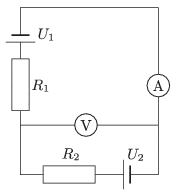# Reading of voltmeter

## Homework Statement

The terminal voltages of the power supplies shown in the figure are constant, and the values of the terminal voltage are: ##U_1=15 V## and ##U_2=45 V##. The resistances of the resistors are ##R_1=10 \Omega## and ##R_2=20 \Omega##.a) What are the readings shown by the meters, which are considered ideal?

b) Solve the problem, if the two meters are swapped over

## The Attempt at a Solution

I have solved part a). I need help with part b).

The following is what I tried.
The circuit for part b) can be drawn as shown in attachment. Since the voltmeter is ideal, no current flows through it, hence, the current flows in the circuit ABCD. The current flowing in ABCD is 45/20=2.25 A which is correct. Since the voltmeter is connected across conducting wires, the reading must be zero but that's not the correct answer. Where did I go wrong?Any help is appreciated. Thanks!

Last edited:

## Answers and Replies

phinds
Science Advisor
Gold Member
You have not reorganized the circuit properly. Try again. Do what it says. Just switch the meters. Do it first without redrawing the circuit and you'll see your error.

•1 person
You have not reorganized the circuit properly. Try again. Do what it says. Just switch the meters. Do it first without redrawing the circuit and you'll see your error.

Hi phinds!I first drew the circuit as is and then removed ##R_1## and ##U_1##. Since they are in series with voltmeter of infinite resistance, they have no role (I think) so I removed them. What do I miss here?

phinds
Science Advisor
Gold Member
You need to rethink why you are removing them. What makes you think they are shorted?

Draw the circuit as is but with the A and V switched, then replace the A with an open circuit and the V with a short circuit and what do you get?

DOH !!!

I meant to say replace the A with a short circuit and the V with an open circuit. Damned dyslexia makes me mix up words sometimes and say one thing when I mean something else, even the opposite.

Last edited:
•1 person
Draw the circuit as is but with the A and V switched, then replace the A with an open circuit and the V with a short circuit and what do you get?
I am not sure but "...replace the A with an open circuit....", is that a typo? We replace A with a conducting wire given that the ammeter is an ideal one, right? I am sorry if I am missing something basic.

I redraw the circuit as shown in attachment. There is no current flowing in ##R_1## so I don't see how that branch is going to affect the potential difference AD.

#### Attachments

I like Serena
Homework Helper
Hey Pranav!You can indeed remove R1 since no current will be flowing.
However, an ideal voltage source will still generate its voltage difference...

•1 person
phinds
Science Advisor
Gold Member
I am not sure but "...replace the A with an open circuit....", is that a typo?

Yes, I corrected my post

Hi ILS! :)

However, an ideal voltage source will still generate its voltage difference...

So what does the voltmeter measure? The potential difference across AD or across the source?I like Serena
Homework Helper
So what does the voltmeter measure? The potential difference across AD or across the source?The potential difference across AD is zero (amp meter counts as short circuit).
The potential difference across R1 is zero (no current = no change in potential).
The potential difference across U1 is 15 V (fixed for an ideal voltage source).
Therefore the voltmeter will measure 15 V.

The potential difference across AD is zero (amp meter counts as short circuit).
The potential difference across R1 is zero (no current = no change in potential).
The potential difference across U1 is 15 V (fixed for an ideal voltage source).
Yes, agreed so far.
Therefore the voltmeter will measure 15 V.
But why? We connect the voltmeter across the wire AD so it should measure zero.I like Serena
Homework Helper
Yes, agreed so far.

But why? We connect the voltmeter across the wire AD so it should measure zero.Huh? I don't think we do.I think we connect the voltmeter to the open ends in your last diagram.
Or if we look at the original diagram, we connect the voltmeter where the amp meter is drawn, which is not AD.

•1 person
Huh? I don't think we do.Sorry, you are right, we don't.But I don't see why it should measure 15 V. Can I look at it by Kirchhoff's law? If I go around the upper loop in my circuit, the potential difference across the voltmeter is 15V so the voltmeter reads 15 V, is this correct?

I like Serena
Homework Helper
Sorry, you are right, we don't.But I don't see why it should measure 15 V. Can I look at it by Kirchhoff's law? If I go around the upper loop in my circuit, the potential difference across the voltmeter is 15V so the voltmeter reads 15 V, is this correct?

Huh? First you say you don't see why it measures 15 V and then you explain why it reads 15 V??Yes, that is the correct application of Kirchhoff's voltage law.

Last edited:
•1 person
Huh? First you say you don't see why it measures 15 V and then you explain that why it reads 15 V??I wasn't sure about my reasoning. Kirchoff's Law didn't hit me the first time I saw the problem.

Thanks a lot ILS!